# GenericMap¶

class sunpy.map.GenericMap(data, header, plot_settings=None, **kwargs)[source]

A Generic spatially-aware 2D data array

Parameters
• data (`numpy.ndarray`, list) – A 2d list or ndarray containing the map data.

• header (dict) – A dictionary of the original image header tags.

• plot_settings (dict, optional) – Plot settings.

• **kwargs – Additional keyword arguments are passed to `NDData` init.

Examples

```>>> import sunpy.map
>>> import sunpy.data.sample
>>> aia = sunpy.map.Map(sunpy.data.sample.AIA_171_IMAGE)
>>> aia
<sunpy.map.sources.sdo.AIAMap object at ...>
SunPy Map
---------
Observatory:                 SDO
Instrument:          AIA 3
Detector:            AIA
Measurement:                 171.0 Angstrom
Wavelength:          171.0 Angstrom
Observation Date:    2011-06-07 06:33:02
Exposure Time:               0.234256 s
Dimension:           [1024. 1024.] pix
Coordinate System:   helioprojective
Scale:                       [2.402792 2.402792] arcsec / pix
Reference Pixel:     [511.5 511.5] pix
Reference Coord:     [3.22309951 1.38578135] arcsec
array([[ -95.92475  ,    7.076416 ,   -1.9656711, ..., -127.96519  ,
-127.96519  , -127.96519  ],
[ -96.97533  ,   -5.1167884,    0.       , ...,  -98.924576 ,
-104.04137  , -127.919716 ],
[ -93.99607  ,    1.0189276,   -4.0757103, ...,   -5.094638 ,
-37.95505  , -127.87541  ],
...,
[-128.01454  , -128.01454  , -128.01454  , ..., -128.01454  ,
-128.01454  , -128.01454  ],
[-127.899666 , -127.899666 , -127.899666 , ..., -127.899666 ,
-127.899666 , -127.899666 ],
[-128.03072  , -128.03072  , -128.03072  , ..., -128.03072  ,
-128.03072  , -128.03072  ]], dtype=float32)
```
```>>> aia.spatial_units
SpatialPair(axis1=Unit("arcsec"), axis2=Unit("arcsec"))
>>> aia.peek()
```

Notes

A number of the properties of this class are returned as two-value named tuples that can either be indexed by position ( or ) or be accessed by the names (.x and .y) or (.axis1 and .axis2). Things that refer to pixel axes use the `.x`, `.y` convention, where x and y refer to the FITS axes (x for columns y for rows). Spatial axes use `.axis1` and `.axis2` which correspond to the first and second axes in the header. `axis1` corresponds to the coordinate axis for `x` and `axis2` corresponds to `y`.

This class makes some assumptions about the WCS information contained in the meta data. The first and most extensive assumption is that it is FITS-like WCS information as defined in the FITS WCS papers.

Within this scope it also makes some other assumptions.

• In the case of APIS convention headers where the CROTAi/j arguments are

provided it assumes that these can be converted to the standard PCi_j notation using equations 32 in Thompson (2006).

• If a CDi_j matrix is provided it is assumed that it can be converted to a

PCi_j matrix and CDELT keywords as described in Greisen & Calabretta (2002)

• The ‘standard’ FITS keywords that are used by this class are the PCi_j

matrix and CDELT, along with the other keywords specified in the WCS papers. All subclasses of this class must convert their header information to this formalism. The CROTA to PCi_j conversion is done in this class.

Warning

This class currently assumes that a header with the CDi_j matrix information also includes the CDELT keywords, without these keywords this class will not process the WCS. Also the rotation_matrix does not work if the CDELT1 and CDELT2 keywords are exactly equal. Also, if a file with more than two dimensions is feed into the class, only the first two dimensions (NAXIS1, NAXIS2) will be loaded and the rest will be discarded.

Attributes Summary

 `bottom_left_coord` The physical coordinate at the center of the bottom left ([0, 0]) pixel. `carrington_latitude` Observer Carrington latitude. `carrington_longitude` Observer Carrington longitude. `center` Return a coordinate object for the center pixel of the array. `cmap` Return the `matplotlib.colors.Colormap` instance this map uses. `coordinate_frame` An `astropy.coordinates.BaseCoordinateFrame` instance created from the coordinate information for this Map, or None if the frame cannot be determined. `coordinate_system` Coordinate system used for x and y axes (ctype1/2). `data` The stored dataset. `date` Image observation time. `detector` Detector name. `dimensions` The dimensions of the array (x axis first, y axis second). `dsun` Observer distance from the center of the Sun. `dtype` The `numpy.dtype` of the array of the map. `exposure_time` Exposure time of the image in seconds. `fits_header` A `Header` representation of the `meta` attribute. `heliographic_latitude` Observer heliographic latitude. `heliographic_longitude` Observer heliographic longitude. `instrument` Instrument name. `latex_name` LaTeX formatted description of the Map. `mask` Mask for the dataset, if any. `measurement` Measurement wavelength. `meta` The map metadata. `name` Human-readable description of the Map. `ndim` The value of `numpy.ndarray.ndim` of the data array of the map. `nickname` An abbreviated human-readable description of the map-type; part of the Helioviewer data model. `observatory` Observatory or Telescope name. `observer_coordinate` The Heliographic Stonyhurst Coordinate of the observer. `processing_level` Returns the FITS processing level if present. `reference_coordinate` Reference point WCS axes in data units (i.e. `reference_pixel` Pixel of reference coordinate. `rotation_matrix` Matrix describing the rotation required to align solar North with the top of the image. `rsun_meters` Radius of the sun in meters. `rsun_obs` Angular radius of the Sun. `scale` Image scale along the x and y axes in units/pixel (i.e. `shifted_value` The total shift applied to the reference coordinate by past applications of `shift`. `size` Deprecated since version 2.1. `spatial_units` Image coordinate units along the x and y axes (i.e. `timeunit` The `Unit` of the exposure time of this observation. `top_right_coord` The physical coordinate at the center of the the top right ([-1, -1]) pixel. `uncertainty` Uncertainty in the dataset, if any. `unit` Unit of the map data. `wavelength` Wavelength of the observation. `waveunit` The `Unit` of the wavelength of this observation. `wcs` the property method being decorated

Methods Summary

 `contour`(level, **kwargs) Returns coordinates of the contours for a given level value. `draw_contours`(levels[, axes]) Draw contours of the data. `draw_grid`([axes, grid_spacing, annotate]) Draws a coordinate overlay on the plot in the Heliographic Stonyhurst coordinate system. `draw_limb`([axes, resolution]) Draws the solar limb as seen by the map’s observer. `draw_quadrangle`(bottom_left, *[, width, …]) Draw a quadrangle defined in world coordinates on the plot using Astropy’s `Quadrangle`. `draw_rectangle`(bottom_left, *[, top_right, …]) Deprecated since version 3.0. `max`(*args, **kwargs) Calculate the maximum value of the data array. `mean`(*args, **kwargs) Calculate the mean of the data array. `min`(*args, **kwargs) Calculate the minimum value of the data array. `peek`([draw_limb, draw_grid, colorbar]) Displays a graphical overview of the data in this object for user evaluation. `pixel_to_world`(x, y[, origin]) Convert a pixel coordinate to a data (world) coordinate. `plot`([annotate, axes, title, autoalign, …]) Plots the map object using matplotlib, in a method equivalent to `imshow()` using nearest neighbor interpolation. Display a quicklook summary of the Map instance using the default web browser. `resample`(dimensions[, method]) Resample to new dimension sizes. `rotate`([angle, rmatrix, order, scale, …]) Returns a new rotated and rescaled map. `save`(filepath[, filetype]) Saves the SunPy Map object to a file. `shift`(axis1, axis2) Returns a map shifted by a specified amount to, for example, correct for a bad map location. `std`(*args, **kwargs) Calculate the standard deviation of the data array. `submap`(bottom_left, *[, top_right, width, …]) Returns a submap defined by a rectangle. `superpixel`(dimensions[, offset, func]) Returns a new map consisting of superpixels formed by applying ‘func’ to the original map data. `world_to_pixel`(coordinate[, origin]) Convert a world (data) coordinate to a pixel coordinate.

Attributes Documentation

bottom_left_coord

The physical coordinate at the center of the bottom left ([0, 0]) pixel.

carrington_latitude

Observer Carrington latitude.

carrington_longitude

Observer Carrington longitude.

center

Return a coordinate object for the center pixel of the array.

If the array has an even number of pixels in a given dimension, the coordinate returned lies on the edge between the two central pixels.

cmap

Return the `matplotlib.colors.Colormap` instance this map uses.

coordinate_frame

An `astropy.coordinates.BaseCoordinateFrame` instance created from the coordinate information for this Map, or None if the frame cannot be determined.

coordinate_system

Coordinate system used for x and y axes (ctype1/2).

If not present, defaults to (HPLN-TAN, HPLT-TAN), and emits a warning.

data

The stored dataset.

Type

`ndarray`-like

date

Image observation time.

This is taken from the ‘DATE-OBS’ FITS keyword.

detector

Detector name.

This is taken from the ‘DETECTOR’ FITS keyword.

dimensions

The dimensions of the array (x axis first, y axis second).

dsun

Observer distance from the center of the Sun.

dtype

The `numpy.dtype` of the array of the map.

exposure_time

Exposure time of the image in seconds.

This is taken from the ‘EXPTIME’ FITS keyword.

fits_header

A `Header` representation of the `meta` attribute.

heliographic_latitude

Observer heliographic latitude.

heliographic_longitude

Observer heliographic longitude.

instrument

Instrument name.

latex_name

LaTeX formatted description of the Map.

mask

Mask for the dataset, if any.

Masks should follow the `numpy` convention that valid data points are marked by `False` and invalid ones with `True`.

Type

any type

measurement

Measurement wavelength.

This is taken from the ‘WAVELNTH’ FITS keywords. If the keyword is not present, defaults to `None`. If ‘WAVEUNIT’ keyword isn’t present, defaults to dimensionless units.

meta

The map metadata.

This is used to intepret the map data. It may have been modified from the original metadata by sunpy. See the `added_items`, `removed_items` and `modified_items` properties of MetaDict to query how the metadata has been modified.

name

Human-readable description of the Map.

ndim

The value of `numpy.ndarray.ndim` of the data array of the map.

nickname

An abbreviated human-readable description of the map-type; part of the Helioviewer data model.

observatory

Observatory or Telescope name.

This is taken from the ‘OBSRVTRY’ FITS keyword.

observer_coordinate

The Heliographic Stonyhurst Coordinate of the observer.

processing_level

Returns the FITS processing level if present.

This is taken from the ‘LVL_NUM’ FITS keyword.

reference_coordinate

Reference point WCS axes in data units (i.e. crval1, crval2). This value includes a shift if one is set.

reference_pixel

Pixel of reference coordinate.

The pixel returned uses zero-based indexing, so will be 1 pixel less than the FITS CRPIX values.

rotation_matrix

Matrix describing the rotation required to align solar North with the top of the image.

The order or precendence of FITS keywords which this is taken from is: - PC*_* - CD*_* - CROTA*

rsun_meters

Radius of the sun in meters.

rsun_obs

Angular radius of the Sun.

Notes

This value is taken the `'rsun_obs'`, `'solar_r'`, or `radius` FITS keywords. If none of these keys are present, the angular radius will be calculated from the radius of the Sun (taken from the `rsun_ref` FITS keyword) and the observer distance. If the `rsun_ref` key is not present, the standard radius of the photosphere will be assumed.

scale

Image scale along the x and y axes in units/pixel (i.e. cdelt1, cdelt2).

shifted_value

The total shift applied to the reference coordinate by past applications of `shift`.

size

Deprecated since version 2.1: Use map.data.size instead

The number of pixels in the array of the map.

spatial_units

Image coordinate units along the x and y axes (i.e. cunit1, cunit2).

timeunit

The `Unit` of the exposure time of this observation.

Taken from the “TIMEUNIT” FITS keyword, and defaults to seconds (as per) the FITS standard).

top_right_coord

The physical coordinate at the center of the the top right ([-1, -1]) pixel.

uncertainty

Uncertainty in the dataset, if any.

Should have an attribute `uncertainty_type` that defines what kind of uncertainty is stored, such as `'std'` for standard deviation or `'var'` for variance. A metaclass defining such an interface is `NDUncertainty` but isn’t mandatory.

Type

any type

unit

Unit of the map data.

This is taken from the ‘BUNIT’ FITS keyword. If no ‘BUNIT’ entry is present in the metadata then this returns `None`. If the ‘BUNIT’ value cannot be parsed into a unit a warning is raised, and `None` returned.

wavelength

Wavelength of the observation.

This is taken from the ‘WAVELNTH’ FITS keywords. If the keyword is not present, defaults to `None`. If ‘WAVEUNIT’ keyword isn’t present, defaults to dimensionless units.

waveunit

The `Unit` of the wavelength of this observation.

This is taken from the ‘WAVEUNIT’ FITS keyword. If the keyword is not present, defaults to `None`

wcs

the property method being decorated

Type

prop

Methods Documentation

contour(level, **kwargs)[source]

Returns coordinates of the contours for a given level value.

For details of the contouring algorithm see `skimage.measure.find_contours`.

Parameters
Returns

contours (list of (n,2) `SkyCoord`) – Coordinates of each contour.

Examples

```>>> import astropy.units as u
>>> import sunpy.map
>>> import sunpy.data.sample
>>> aia = sunpy.map.Map(sunpy.data.sample.AIA_171_IMAGE)
>>> contours = aia.contour(50000 * u.ct)
>>> print(contours)
<SkyCoord (Helioprojective: obstime=2011-06-07T06:33:02.770, rsun=696000.0 km, observer=<HeliographicStonyhurst Coordinate (obstime=2011-06-07T06:33:02.770, rsun=696000.0 km): (lon, lat, radius) in (deg, deg, m)
(-0.00406308, 0.04787238, 1.51846026e+11)>): (Tx, Ty) in arcsec
[(719.59798458, -352.60839064), (717.19243987, -353.75348121),
...
```
draw_contours(levels, axes=None, **contour_args)[source]

Draw contours of the data.

Parameters
Returns

cs (`list`) – The `QuadContourSet` object, after it has been added to `axes`.

Notes

Extra keyword arguments to this function are passed through to the `contour` function.

draw_grid(axes=None, grid_spacing: Unit("deg") = <Quantity 15. deg>, annotate=True, **kwargs)[source]

Draws a coordinate overlay on the plot in the Heliographic Stonyhurst coordinate system.

To overlay other coordinate systems see the WCSAxes Documentation

Parameters
Returns

overlay (`CoordinatesMap`) – The wcsaxes coordinate overlay instance.

Notes

Keyword arguments are passed onto the `sunpy.visualization.wcsaxes_compat.wcsaxes_heliographic_overlay` function.

draw_limb(axes=None, *, resolution=1000, **kwargs)[source]

Draws the solar limb as seen by the map’s observer.

The limb is a circle for only the simplest plots. If the coordinate frame of the limb is different from the coordinate frame of the plot axes, not only may the limb not be a true circle, a portion of the limb may be hidden from the observer. In that case, the circle is divided into visible and hidden segments, represented by solid and dotted lines, respectively.

Parameters
• axes (`axes` or `None`) – Axes to plot limb on or `None` to use current axes.

• resolution (`int`) – The number of points to use to represent the limb.

Returns

Notes

Keyword arguments are passed onto the patches.

If the limb is a true circle, `visible` will instead be `Circle` and `hidden` will be `None`.

To avoid triggering Matplotlib auto-scaling, these patches are added as artists instead of patches. One consequence is that the plot legend is not populated automatically when the limb is specified with a text label. See Composing Custom Legends in the Matplotlib documentation for examples of creating a custom legend.

draw_quadrangle(bottom_left, *, width: Unit(‘deg’) = None, height: Unit(‘deg’) = None, axes=None, top_right=None, **kwargs)[source]

Draw a quadrangle defined in world coordinates on the plot using Astropy’s `Quadrangle`.

This draws a quadrangle that has corners at `(bottom_left, top_right)`, and has sides aligned with the coordinate axes of the frame of `bottom_left`, which may be different from the coordinate axes of the map.

If `width` and `height` are specified, they are respectively added to the longitude and latitude of the `bottom_left` coordinate to calculate a `top_right` coordinate.

Parameters
Returns

quad (`Quadrangle`) – The added patch

Notes

Extra keyword arguments to this function are passed through to the `Quadrangle` instance.

Examples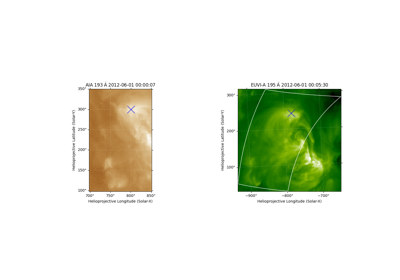AIA to STEREO coordinate conversion¶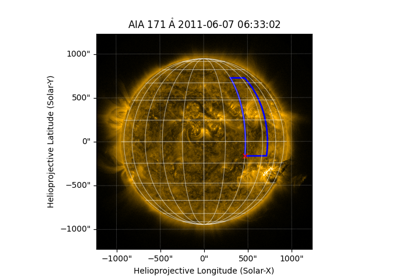Drawing a latitude-longitude quadrangle¶Drawing a rectangle on a map¶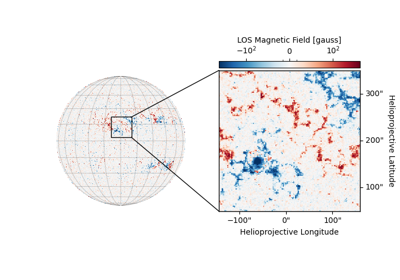HMI Showcase: Cutout¶
draw_rectangle(bottom_left, *, top_right=None, width: Unit(‘deg’) = None, height: Unit(‘deg’) = None, axes=None, **kwargs)[source]

Deprecated since version 3.0: The draw_rectangle function is deprecated and may be removed in version 3.1. Use draw_quadrangle instead.

Draw a rectangle defined in world coordinates on the plot.

This draws a rectangle that has corners at `(bottom_left, top_right)`, and has sides parallel to coordinate axes of the map.

If `width` and `height` are specified, they are respectively added to the longitude and latitude of the `bottom_left` coordinate to calculate a `top_right` coordinate.

Parameters
Returns

rect (`list`) – A list containing the `Rectangle` object, after it has been added to `axes`.

Notes

Extra keyword arguments to this function are passed through to the `Rectangle` instance.

max(*args, **kwargs)[source]

Calculate the maximum value of the data array.

mean(*args, **kwargs)[source]

Calculate the mean of the data array.

min(*args, **kwargs)[source]

Calculate the minimum value of the data array.

peek(draw_limb=False, draw_grid=False, colorbar=True, **matplot_args)[source]

Displays a graphical overview of the data in this object for user evaluation. For the creation of plots, users should instead use the `plot` method and Matplotlib’s pyplot framework.

Parameters
• draw_limb (bool) – Whether the solar limb should be plotted.

• draw_grid (bool or `Quantity`) – Whether solar meridians and parallels are plotted. If `Quantity` then sets degree difference between parallels and meridians.

• colorbar (bool) – Whether to display a colorbar next to the plot.

• **matplot_args (dict) – Matplotlib Any additional imshow arguments that should be used when plotting.

pixel_to_world(x: Unit(‘pix’), y: Unit(‘pix’), origin=None)[source]

Convert a pixel coordinate to a data (world) coordinate.

Parameters
• x (`Quantity`) – Pixel coordinate of the CTYPE1 axis. (Normally solar-x).

• y (`Quantity`) – Pixel coordinate of the CTYPE2 axis. (Normally solar-y).

• origin (int) –

Deprecated.

Origin of the top-left corner. i.e. count from 0 or 1. Normally, origin should be 0 when passing numpy indices, or 1 if passing values from FITS header or map attributes.

Returns

coord (`astropy.coordinates.SkyCoord`) – A coordinate object representing the output coordinate.

plot(annotate=True, axes=None, title=True, autoalign=False, clip_interval: Unit(‘%’) = None, **imshow_kwargs)[source]

Plots the map object using matplotlib, in a method equivalent to `imshow()` using nearest neighbor interpolation.

Parameters

Examples

```>>> # Simple Plot with color bar
>>> aia.plot()
>>> plt.colorbar()
```
```>>> # Add a limb line and grid
>>> aia.plot()
>>> aia.draw_limb()
>>> aia.draw_grid()
```

Notes

The `autoalign` functionality is computationally intensive. If the plot will be interactive, the alternative approach of preprocessing the map (e.g., de-rotating it) to match the desired axes will result in better performance.

When combining `autoalign` functionality with `Helioprojective` coordinates, portions of the map that are beyond the solar disk may not appear, which may also inhibit Matplotlib’s autoscaling of the plot limits. The plot limits can be set manually. To preserve the off-disk parts of the map, using the `assume_spherical_screen()` context manager may be appropriate.

quicklook()[source]

Display a quicklook summary of the Map instance using the default web browser.

Notes

The image colormap uses histogram equalization.

Clicking on the image to switch between pixel space and coordinate space requires Javascript support to be enabled in the web browser.

Examples

```>>> from sunpy.map import Map
>>> import sunpy.data.sample
>>> smap = Map(sunpy.data.sample.AIA_171_IMAGE)
>>> smap.quicklook()
```

(which will open the following content in the default web browser)

`<sunpy.map.sources.sdo.AIAMap object at 0x7f9c94c3bd30>`
Observatory SDO AIA 3 AIA 171.0 Angstrom 171.0 Angstrom 2011-06-07 06:33:02 0.234256 s [1024. 1024.] pix helioprojective [2.402792 2.402792] arcsec / pix [511.5 511.5] pix [3.22309951 1.38578135] arcsec
Image colormap uses histogram equalization
Click on the image to toggle between units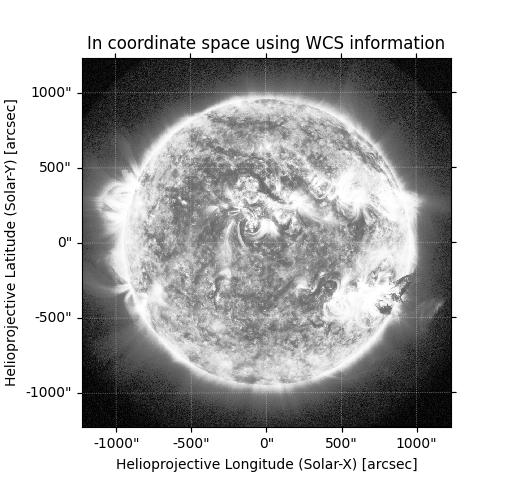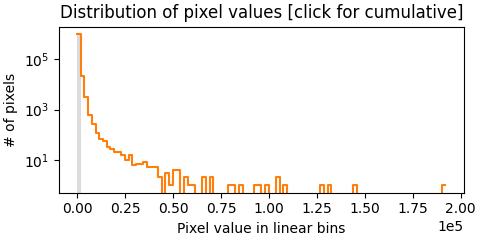resample(dimensions: Unit(‘pix’), method='linear')[source]

Resample to new dimension sizes.

Uses the same parameters and creates the same co-ordinate lookup points as IDL’’s congrid routine, which apparently originally came from a VAX/VMS routine of the same name.

Parameters
• dimensions (`Quantity`) – Output pixel dimensions. The first argument corresponds to the ‘x’ axis and the second argument corresponds to the ‘y’ axis.

• method (str) –

Method to use for resampling interpolation.

Returns

out (`GenericMap` or subclass) – Resampled map

References

Rebinning

rotate(angle: Unit(‘deg’) = None, rmatrix=None, order=4, scale=1.0, recenter=False, missing=0.0, use_scipy=False)[source]

Returns a new rotated and rescaled map.

Specify either a rotation angle or a rotation matrix, but not both. If neither an angle or a rotation matrix are specified, the map will be rotated by the rotation angle in the metadata.

The map will be rotated around the reference coordinate defined in the meta data.

This method also updates the `rotation_matrix` attribute and any appropriate header data so that they correctly describe the new map.

Parameters
• angle (`Quantity`) – The angle (degrees) to rotate counterclockwise.

• rmatrix (array-like) – 2x2 linear transformation rotation matrix.

• order (int) – Interpolation order to be used. Must be in the range 0-5. When using scikit-image this parameter is passed into `skimage.transform.warp()` (e.g., 4 corresponds to bi-quartic interpolation). When using scipy it is passed into `scipy.ndimage.affine_transform()` where it controls the order of the spline. Faster performance may be obtained at the cost of accuracy by using lower values. Default: 4

• scale (float) – A scale factor for the image, default is no scaling

• recenter (bool) – If True, position the axis of rotation at the center of the new map Default: False

• missing (float) – The numerical value to fill any missing points after rotation. Default: 0.0

• use_scipy (bool) – If True, forces the rotation to use `scipy.ndimage.affine_transform()`, otherwise it uses the `skimage.transform.warp()`. Default: False, unless scikit-image can’t be imported

Returns

out (`GenericMap` or subclass) – A new Map instance containing the rotated and rescaled data of the original map.

See also

`sunpy.image.transform.affine_transform`

The routine this method calls for the rotation.

Notes

This function will remove old CROTA keywords from the header. This function will also convert a CDi_j matrix to a PCi_j matrix.

See `sunpy.image.transform.affine_transform()` for details on the transformations, situations when the underlying data is modified prior to rotation, and differences from IDL’s rot().

save(filepath, filetype='auto', **kwargs)[source]

Saves the SunPy Map object to a file.

Currently SunPy can only save files in the FITS format. In the future support will be added for saving to other formats.

Parameters
shift(axis1: Unit(‘deg’), axis2: Unit(‘deg’))[source]

Returns a map shifted by a specified amount to, for example, correct for a bad map location. These values are applied directly to the `reference_coordinate`. To check how much shift has already been applied see `shifted_value`

Parameters
Returns

out (`GenericMap` or subclass) – A new shifted Map.

std(*args, **kwargs)[source]

Calculate the standard deviation of the data array.

submap(bottom_left, *, top_right=None, width: (Unit(‘deg’), Unit(‘pix’)) = None, height: (Unit(‘deg’), Unit(‘pix’)) = None)[source]

Returns a submap defined by a rectangle.

Any pixels which have at least part of their area inside the rectangle are returned. If the rectangle is defined in world coordinates, the smallest array which contains all four corners of the rectangle as defined in world coordinates is returned.

Parameters
Returns

out (`GenericMap` or subclass) – A new map instance is returned representing to specified sub-region.

Notes

When specifying pixel coordinates, they are specified in Cartesian order not in numpy order. So, for example, the `bottom_left=` argument should be `[left, bottom]`.

Examples

```>>> import astropy.units as u
>>> from astropy.coordinates import SkyCoord
>>> import sunpy.map
>>> import sunpy.data.sample
>>> aia = sunpy.map.Map(sunpy.data.sample.AIA_171_IMAGE)
>>> bl = SkyCoord(-300*u.arcsec, -300*u.arcsec, frame=aia.coordinate_frame)
>>> tr = SkyCoord(500*u.arcsec, 500*u.arcsec, frame=aia.coordinate_frame)
>>> aia.submap(bl, top_right=tr)
<sunpy.map.sources.sdo.AIAMap object at ...>
SunPy Map
---------
Observatory:         SDO
Instrument:          AIA 3
Detector:            AIA
Measurement:         171.0 Angstrom
Wavelength:          171.0 Angstrom
Observation Date:    2011-06-07 06:33:02
Exposure Time:       0.234256 s
Dimension:           [335. 335.] pix
Coordinate System:   helioprojective
Scale:               [2.402792 2.402792] arcsec / pix
Reference Pixel:     [126.5 125.5] pix
Reference Coord:     [3.22309951 1.38578135] arcsec
...
```
```>>> aia.submap([0,0]*u.pixel, top_right=[5,5]*u.pixel)
<sunpy.map.sources.sdo.AIAMap object at ...>
SunPy Map
---------
Observatory:         SDO
Instrument:          AIA 3
Detector:            AIA
Measurement:         171.0 Angstrom
Wavelength:          171.0 Angstrom
Observation Date:    2011-06-07 06:33:02
Exposure Time:       0.234256 s
Dimension:           [6. 6.] pix
Coordinate System:   helioprojective
Scale:               [2.402792 2.402792] arcsec / pix
Reference Pixel:     [511.5 511.5] pix
Reference Coord:     [3.22309951 1.38578135] arcsec
...
```
```>>> width = 10 * u.arcsec
>>> height = 10 * u.arcsec
>>> aia.submap(bl, width=width, height=height)
<sunpy.map.sources.sdo.AIAMap object at ...>
SunPy Map
---------
Observatory:         SDO
Instrument:          AIA 3
Detector:            AIA
Measurement:         171.0 Angstrom
Wavelength:          171.0 Angstrom
Observation Date:    2011-06-07 06:33:02
Exposure Time:       0.234256 s
Dimension:           [5. 5.] pix
Coordinate System:   helioprojective
Scale:               [2.402792 2.402792] arcsec / pix
Reference Pixel:     [125.5 125.5] pix
Reference Coord:     [3.22309951 1.38578135] arcsec
...
```
```>>> bottom_left_vector = SkyCoord([0, 10]  * u.deg, [0, 10] * u.deg, frame='heliographic_stonyhurst')
>>> aia.submap(bottom_left_vector)
<sunpy.map.sources.sdo.AIAMap object at ...>
SunPy Map
---------
Observatory:         SDO
Instrument:          AIA 3
Detector:            AIA
Measurement:         171.0 Angstrom
Wavelength:          171.0 Angstrom
Observation Date:    2011-06-07 06:33:02
Exposure Time:       0.234256 s
Dimension:           [70. 69.] pix
Coordinate System:   helioprojective
Scale:               [2.402792 2.402792] arcsec / pix
Reference Pixel:     [1.5 0.5] pix
Reference Coord:     [3.22309951 1.38578135] arcsec
...
```
superpixel(dimensions: Unit("pix"), offset: Unit("pix") = <Quantity [0., 0.] pix>, func=<function sum>)[source]

Returns a new map consisting of superpixels formed by applying ‘func’ to the original map data.

Parameters
• dimensions (tuple) – One superpixel in the new map is equal to (dimension, dimension) pixels of the original map. The first argument corresponds to the ‘x’ axis and the second argument corresponds to the ‘y’ axis. If non-integer values are provided, they are rounded using `int()`.

• offset (tuple) – Offset from (0,0) in original map pixels used to calculate where the data used to make the resulting superpixel map starts. If non-integer value are provided, they are rounded using `int()`.

• func – Function applied to the original data. The function ‘func’ must take a numpy array as its first argument, and support the axis keyword with the meaning of a numpy axis keyword (see the description of `sum` for an example.) The default value of ‘func’ is `sum`; using this causes superpixel to sum over (dimension, dimension) pixels of the original map.

Returns

out (`GenericMap` or subclass) – A new Map which has superpixels of the required size.

References

world_to_pixel(coordinate, origin=None)[source]

Convert a world (data) coordinate to a pixel coordinate.

Parameters
• coordinate (`SkyCoord` or `BaseCoordinateFrame`) – The coordinate object to convert to pixel coordinates.

• origin (int) –

Deprecated.

Origin of the top-left corner. i.e. count from 0 or 1. Normally, origin should be 0 when passing numpy indices, or 1 if passing values from FITS header or map attributes.

Returns